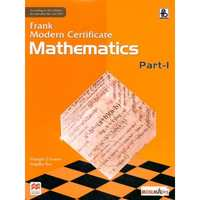# Frank solutions for Class 9 Maths ICSE chapter 20 - Constructions of Quadrilaterals [Latest edition]

#### Chapters## Chapter 20: Constructions of Quadrilaterals

Exercise 20.1
Exercise 20.1

### Frank solutions for Class 9 Maths ICSE Chapter 20 Constructions of Quadrilaterals Exercise 20.1

Exercise 20.1 | Q 1.1

Construct a quadrilateral ABCD in which
AB = 4.6cm, BD = 5cm, AC = 6cm, CD = 4.2cm and ∠A = 90°

Exercise 20.1 | Q 1.2

Construct a quadrilateral ABCD in which
AB = 7.2cm, BC = 5.8cm, CD = 6.3cm, AD = 4.3cm and ∠A = 75°

Exercise 20.1 | Q 1.3

Construct a quadrilateral ABCD in which
AB = 4.8cm, AC = 5.8cm, AD = 3.6cm, ∠A = 105° and ∠B = 60°

Exercise 20.1 | Q 1.4

Construct a quadrilateral ABCD in which
AD = AB = 5cm, BC = 3.8cm, CD = 3.5cm, and ∠BAD = 45°

Exercise 20.1 | Q 2.1

Construct a trapezium ABCD in which
AB = 3.5cm, BC = 6cm, CD = 3.5cm, AD = 4.4cm and AD || BC.

Exercise 20.1 | Q 2.2

Construct a trapezium ABCD in which
AB = 4.6cm, BC = 6.4cm, CD = 5.6cm, ∠B = 60° and AD || BC.

Exercise 20.1 | Q 3.1

Construct a parallelogram ABCD. Given:
AB = 5.5cm, BC = 3.5cm, ∠B = 75°

Exercise 20.1 | Q 3.2

Construct a parallelogram ABCD. Given:
AB = 3.5cm, AC = 4cm and BD = 5.2cm

Exercise 20.1 | Q 4

Construct a parallelogram PQRS in which PQ = 6.4cm, QR = 4cm and the distance between PQ and SR is 3cm.

Exercise 20.1 | Q 5

Construct a parallelogram ABCD in which AB = 4.5cm, ∠A = 105° and the distance between AB and CD is 3.2cm.

Exercise 20.1 | Q 6

Construct a rhombus ABCD, given AB = 3.8cm and ∠A = 60°. Measure AC.

Exercise 20.1 | Q 7

Construct a rhombus whose perimeter is 16cm and BD = 6.2cm.

Exercise 20.1 | Q 8

Construct a rhombus whose diagonals AC = 7.4cm and BD = 6cm.

Exercise 20.1 | Q 9

Construct a rhombus whose side AB = 5cm and diagonal AC = 6cm. MEasure DB and AD.

Exercise 20.1 | Q 10

Construct a square with each side 4.3cm

Exercise 20.1 | Q 11

Construct a square ABCD with AC = 6.5cm

Exercise 20.1 | Q 12

Construct a square with perimeter = 18cm.

Exercise 20.1 | Q 13

Construct a square whose area is 25 sq. cm.

Exercise 20.1 | Q 14.1

Construct a rectangle ABCD, when AD = 3.2cm and diagonal BD = 5.5cm. Measure CD.

Exercise 20.1 | Q 14.2

Construct a rectangle ABCD, when AB = 5cm and BC = 6.2cm.

Exercise 20.1 | Q 15

Construct a rectangle ABCD with one diagonal AC = 5.8cm and the acute angle between the diagonals is equal to 45°.

Exercise 20.1 | Q 16

Construct a rectangle ABCD with perimeter 18cm and AB = 6cm.

Exercise 20.1 | Q 17

Construct a rectangle ABCCD, AB = 6cm. ∠CAB = 30°.

Exercise 20.1 | Q 18.1

Construct a rectangle PQRS, when its Area = 21 cm2 and length = 4.2cm

Exercise 20.1 | Q 18.2

Construct a rectangle PQRS, when its Area = 33.8cm2 and breadth = 6.5cm

Exercise 20.1 | Q 19

Construct a regular hexagon of side 3.5cm

Exercise 20.1 | Q 20

Construct a regular hexagon of side 4cm.

## Chapter 20: Constructions of Quadrilaterals

Exercise 20.1## Frank solutions for Class 9 Maths ICSE chapter 20 - Constructions of Quadrilaterals

Frank solutions for Class 9 Maths ICSE chapter 20 (Constructions of Quadrilaterals) include all questions with solution and detail explanation. This will clear students doubts about any question and improve application skills while preparing for board exams. The detailed, step-by-step solutions will help you understand the concepts better and clear your confusions, if any. Shaalaa.com has the CISCE Class 9 Maths ICSE solutions in a manner that help students grasp basic concepts better and faster.

Further, we at Shaalaa.com provide such solutions so that students can prepare for written exams. Frank textbook solutions can be a core help for self-study and acts as a perfect self-help guidance for students.

Concepts covered in Class 9 Maths ICSE chapter 20 Constructions of Quadrilaterals are Construction of Parallelograms, Construction of Trapezium, Construction of a Rectangle When Its Length and Breadth Are Given., Construction of Rhombus, To Construct a Regular Hexagon, Constructing a Quadrilateral, Construction of Square.

Using Frank Class 9 solutions Constructions of Quadrilaterals exercise by students are an easy way to prepare for the exams, as they involve solutions arranged chapter-wise also page wise. The questions involved in Frank Solutions are important questions that can be asked in the final exam. Maximum students of CISCE Class 9 prefer Frank Textbook Solutions to score more in exam.

Get the free view of chapter 20 Constructions of Quadrilaterals Class 9 extra questions for Class 9 Maths ICSE and can use Shaalaa.com to keep it handy for your exam preparation